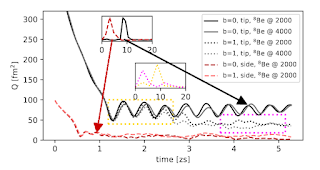## Tuesday, 7 January 2020

### A new relativistic nuclear dynamics code

I note a new paper in press in Physics Letters B by Z. X. Ren et al. (doi: 10.1016/j.physletb.2019.135194) which presents new calculations of alpha cluster dynamics using a new code developed using relativistic density functional theory.  This is a method very similar to much of my research, and it's great that the authors have a production code using the relatvistic theory (my work is non-relativistic).

This paper on alpha cluster dynamics looks at overlapping problems to my last paper on the subject.  I note that mine was submitted on 05/09/2019 while theirs on 09/09/2019 :-) and it's good to see different methods being used for the same kind of thing.  Our plots of the Fourier strength of the quadrupole vibrations in the Be-8+alpha system ought to be directly comparable:Spectral power for quadrupole resonances in He-4 + Be-8 system from Ren et al. (in press) Insets show spectral density for He-4 + Be-8 quadrupole vibrations (x-axis units are MeV) from Stevenson et al. (in press)

In the Ren at all work, then solid peak should correspond to the same physical quantity as (probably) the solid peak in my work in the upper inset in the right figure.  Ren's units give the period of the vibration in fm/c, while I give energy in MeV.  The peak from Ren's calculation has a period of ~120 fm/c.  These strange time units are the amount of time it takes light to travel 1 femtometre (10-15 m).  That's a very short amount of time (10-15 / 3×108 ≅ 3.33 × 10-24 s = 3.33 ys = 0.0033 zs).  So 120 fm/c is 0.4 zs (zeptoseconds).  The frequency (number of such vibrations per second) is f=1/0.4 zs which is 2.5×1021 s-1.

According to Planck's quantization rule, these vibrations have a corresponding energy E=hf, where h is Planck's constant.  so E = (4×10-21 MeV s)(2.5×1021 s-1) ~ 10 MeV, so we appear to agree with each other pretty well.  That's not really surprising, since the nuclear forces we use have to be reasonable enough to reproduce the right kind of scale of nuclear properties, but it's still reassuring.